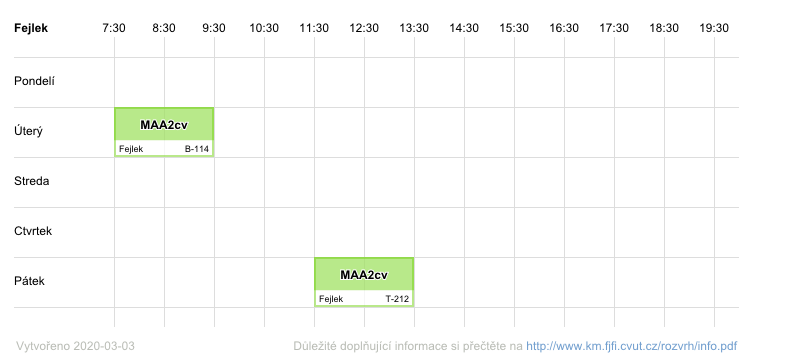# Ing. Jiří Fejlek (doktorand)

 e-mail: zobrazit e-mail

 školitel: doc. Dr. techn. Stefan Ratschan, Dipl.-Ing. zahájení studia: 01.03.2018 forma studia: prezenční téma disertační práce: Optimal Control with Adversarial Events popis: Assume an ordinary differential equation with inputs. Classical optimal control is the problem of finding inputs such that resulting solution of the differential equation optimizes a given objective function. The thesis will study an extension of this problem where some random events beyond our control can influence the differential equation, and the goal is to optimize the expected value of the objective function. This amounts to optimal control for a certain class of stochastic hybrid dynamical systems . The goal of this thesis topic will be the design of an algorithm for solving this problem that can efficiently solve benchmark problems of a size that goes beyond the capabilities of current methods. Suggested basic ingredients will be: - Multiple shooting: a method that can solve highly non-trivial classical optimal control problems , but creates inputs that are not able to react to external events. - Approximate dynamic programming : a method that efficiently solves the optimal control problem for Markov decision processes, which corresponds to the given problem, but without the presence of differential equations.za obsah této stránky zodpovídá: Radek Fučík | naposledy změněno: 15.8.2011
Trojanova 13, 120 00 Praha 2, tel. 224 358 540, pevná linka 224 923 098, fax 234 358 643